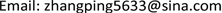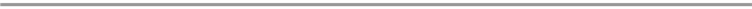﻿ 新的双辅助微分方程展开法求色散水波方程的精确解 A New Double-Auxiliary Differential Equation Expansion Method for Exact Solutions of Dispersive Water Wave Equations

Vol. 09  No. 03 ( 2020 ), Article ID: 34628 , 6 pages
10.12677/AAM.2020.93044

A New Double-Auxiliary Differential Equation Expansion Method for Exact Solutions of Dispersive Water Wave Equations

Ping Zhang1, Yuhuai Sun2, Feng Luo3

1The Engineering & Technical College of Chengdu University of Technology, Leshan Sichuan

2Sichuan Normal University, Chengdu Sichuan

3Leshan Vocational & Technical College, Leshan SichuanReceived: Feb. 29th, 2020; accepted: Mar. 13th, 2020; published: Mar. 20th, 2020ABSTRACT

Based on the existing auxiliary differential equations and G'/G-expansion method, this paper constructs a new double auxiliary differential equation expansion method, and uses the double auxiliary differential equation expansion method to solve new exact solutions of dispersive water wave equations: hyperbolic function formal solution, rational function formal solution, trigonometric function formal solution, hyperbolic function and trigonometric function mixed form solution, trigonometric function and rational function mixed form solution. The method can also be applied to solve other nonlinear equations.

Keywords:Double Auxiliary Equation Expansion Method, Homogeneous Equilibrium Method, Dispersive Water Wave Equation, Exact Solution1成都理工大学工程技术学院，四川 乐山

2四川师范大学，四川 成都

3乐山职业技术学院，四川 乐山1. 引言

2. 双辅助方程展开法

$\left\{\begin{array}{l}P\left(u,v,{u}_{t},{v}_{t},{u}_{x},{v}_{x},\cdots \right)=0\\ H\left(u,v,{u}_{t},{v}_{t},{u}_{x},{v}_{x},\cdots \right)=0\end{array}$ (1)

$\left\{\begin{array}{l}u\left(x,t\right)=\underset{k=0}{\overset{m}{\sum }}\underset{i+j=k}{\sum }{a}_{ij}\left(x,t\right){\varphi }^{i}\left(\xi \right){\left(\frac{{G}^{\prime }\left(\theta \right)}{G\left(\theta \right)}\right)}^{j},\\ v\left(x,t\right)=\underset{k=0}{\overset{n}{\sum }}\underset{i+j=k}{\sum }{b}_{ij}\left(x,t\right){\varphi }^{i}\left(\xi \right){\left(\frac{{G}^{\prime }\left(\theta \right)}{G\left(\theta \right)}\right)}^{j},\end{array}$ (2)

${\varphi }^{\prime }\left(\xi \right)=A+B\varphi \left(\xi \right)+C{\varphi }^{2}\left(\xi \right)$ (3)

${G}^{″}\left(\theta \right)+\lambda {G}^{\prime }\left(\theta \right)+\mu G\left(\theta \right)=0$ (4)

${\varphi }_{1}\left(\xi \right)=-\frac{B+\sqrt{\Delta }}{2C}\mathrm{tanh}\left(\frac{\sqrt{\Delta }}{2}\xi \right)$ (5)

${\varphi }_{2}\left(\xi \right)=-\frac{B+\sqrt{\Delta }}{2C}\mathrm{coth}\left(\frac{\sqrt{\Delta }}{2}\xi \right)$ (6)

$\Delta ={B}^{2}-4AC<0$ 时，方程(3)的解为：

${\varphi }_{3}\left(\xi \right)=-\frac{B-\sqrt{-\Delta }}{2C}\mathrm{tan}\left(\frac{\sqrt{-\Delta }}{2}\xi \right)$ (7)

${\varphi }_{4}\left(\xi \right)=-\frac{B-\sqrt{-\Delta }}{2C}\mathrm{cot}\left(\frac{\sqrt{-\Delta }}{2}\xi \right)$ (8)

$A=B=0,C\ne 0$ 时，方程(3)的解为：

${\varphi }_{5}\left(\xi \right)=\frac{-1}{C\xi +{H}_{1}}$ (9)

${\Delta }_{1}={\lambda }^{2}-4\mu >0$ 时，方程(4)的解为：

$\frac{{G}^{\prime }\left(\theta \right)}{G\left(\theta \right)}=-\frac{\lambda }{2}+\frac{\sqrt{{\Delta }_{1}}}{2}\frac{{c}_{1}\mathrm{sinh}\left(\frac{\sqrt{{\Delta }_{1}}}{2}\theta \right)+{c}_{2}\mathrm{cosh}\left(\frac{\sqrt{{\Delta }_{1}}}{2}\theta \right)}{{c}_{1}\mathrm{cosh}\left(\frac{\sqrt{{\Delta }_{1}}}{2}\theta \right)+{c}_{2}\mathrm{sinh}\left(\frac{\sqrt{{\Delta }_{1}}}{2}\theta \right)}$ (10)

${\Delta }_{1}={\lambda }^{2}-4\mu <0$ 时，方程(4)的解为：

$\frac{{G}^{\prime }\left(\theta \right)}{G\left(\theta \right)}=-\frac{\lambda }{2}+\frac{\sqrt{-{\Delta }_{1}}}{2}\frac{-{c}_{1}\mathrm{sin}\left(\frac{\sqrt{-{\Delta }_{1}}}{2}\theta \right)+{c}_{2}\mathrm{cos}\left(\frac{\sqrt{-{\Delta }_{1}}}{2}\theta \right)}{{c}_{1}\mathrm{cos}\left(\frac{\sqrt{-{\Delta }_{1}}}{2}\theta \right)+{c}_{2}\mathrm{sin}\left(\frac{\sqrt{-{\Delta }_{1}}}{2}\theta \right)}$ (11)

${\Delta }_{1}={\lambda }^{2}-4\mu =0$ 时，方程(4)的解为：

$\frac{{G}^{\prime }\left(\theta \right)}{G\left(\theta \right)}=-\frac{\lambda }{2}+\frac{{c}_{2}}{{c}_{1}+{c}_{2}\theta }$ (12)

3. 色散水波方程的解

$\left\{\begin{array}{l}{u}_{t}={v}_{xxx}+2{\left(uv\right)}_{x}=0\\ {v}_{t}={u}_{x}+2v{v}_{x}=0\end{array}$ (13)

$\left\{\begin{array}{l}u\left(x,t\right)={a}_{0}\left(t\right)+{a}_{1}\left(t\right)\varphi \left(\xi \right)+{a}_{2}\left(t\right)\frac{{G}^{\prime }\left(\theta \right)}{G\left(\theta \right)}+{a}_{3}\left(t\right)\varphi \left(\xi \right)\frac{{G}^{\prime }\left(\theta \right)}{G\left(\theta \right)}\\ v\left(x,t\right)={b}_{0}\left(t\right)+{b}_{1}\left(t\right)\varphi \left(\xi \right)+{b}_{2}\left(t\right)\frac{{G}^{\prime }\left(\theta \right)}{G\left(\theta \right)}\end{array}$ (14)

${\varphi }^{i}\left(\xi \right){\left(\frac{{G}^{\prime }\left(\theta \right)}{G\left(\theta \right)}\right)}^{j}\left(i,j=0,1,2,3\right)$ 的多项式，令每一项幂系数为零，得到一个关于 ${a}_{0}\left(t\right),{a}_{1}\left(t\right),{a}_{2}\left(t\right),{b}_{0}\left(t\right),{b}_{1}\left(t\right),$

${b}_{2}\left(t\right),{k}_{1},{k}_{2},{\varpi }_{1}$${\varpi }_{2}$ 的线性方程组，借助Maple求解此方程组，可得到：

${k}_{1}={k}_{1},{k}_{2}=\frac{{k}_{1}B}{\lambda }$

${\varpi }_{1}=1,{\varpi }_{2}=\frac{B}{\lambda }$

${a}_{0}\left(t\right)=\frac{1}{4}\frac{{k}_{2}^{2}\lambda \left(1+5\mu \right)}{\mu },{a}_{1}\left(t\right)=-\frac{{b}_{2}{k}_{2}^{3}\lambda \mu \left(1+5\mu \right)}{4\mu {b}_{0}{k}_{1}A}$ (15)

${a}_{2}\left(t\right)=-\frac{1}{4}\frac{{b}_{2}{k}_{2}^{2}\lambda \left(1+5\mu \right)}{\mu {b}_{0}},{a}_{3}\left(t\right)=\frac{{b}_{2}{k}_{2}^{3}{\lambda }^{2}\left(1+5\mu \right)}{4\mu {b}_{0}{k}_{1}A}$

${b}_{0}\left(t\right)={b}_{0}\left(t\right),{b}_{1}\left(t\right)={b}_{1}\left(t\right),{b}_{2}\left(t\right)={b}_{2}\left(t\right)$

$\begin{array}{c}{u}_{1}\left(x,t\right)=\frac{1}{4}\frac{{k}_{2}^{2}\lambda \left(1+5\mu \right)}{\mu }-\frac{{b}_{2}{k}_{2}^{3}\lambda \mu \left(1+5\mu \right)}{4\mu {b}_{0}{k}_{1}A}\frac{B+\sqrt{\Delta }}{2C}\mathrm{coth}\left(\frac{\sqrt{\Delta }}{2}\xi \right)\\ \text{\hspace{0.17em}}\text{\hspace{0.17em}}+\frac{1}{4}\frac{{b}_{2}{k}_{2}^{2}\lambda \left(1+5\mu \right)}{\mu {b}_{0}}\left(\frac{\lambda }{2}-\frac{\sqrt{{\Delta }_{1}}}{2}\frac{{c}_{1}\mathrm{sinh}\left(\frac{\sqrt{{\Delta }_{1}}}{2}\theta \right)+{c}_{2}\mathrm{cosh}\left(\frac{\sqrt{{\Delta }_{1}}}{2}\theta \right)}{{c}_{1}\mathrm{cosh}\left(\frac{\sqrt{{\Delta }_{1}}}{2}\theta \right)+{c}_{2}\mathrm{sinh}\left(\frac{\sqrt{{\Delta }_{1}}}{2}\theta \right)}\right)\\ \text{\hspace{0.17em}}\text{\hspace{0.17em}}+\frac{{b}_{2}{k}_{2}^{3}{\lambda }^{2}\left(1+5\mu \right)}{4\mu {b}_{0}{k}_{1}A}\frac{B+\sqrt{\Delta }}{2C}\mathrm{coth}\left(\frac{\sqrt{\Delta }}{2}\xi \right)\left(\frac{\lambda }{2}-\frac{\sqrt{{\Delta }_{1}}}{2}\frac{{c}_{1}\mathrm{sinh}\left(\frac{\sqrt{{\Delta }_{1}}}{2}\theta \right)+{c}_{2}\mathrm{cosh}\left(\frac{\sqrt{{\Delta }_{1}}}{2}\theta \right)}{{c}_{1}\mathrm{cosh}\left(\frac{\sqrt{{\Delta }_{1}}}{2}\theta \right)+{c}_{2}\mathrm{sinh}\left(\frac{\sqrt{{\Delta }_{1}}}{2}\theta \right)}\right)\end{array}$ (16)

$\begin{array}{c}{v}_{1}\left(x,t\right)={b}_{0}\left(t\right)-{b}_{1}\left(t\right)\frac{B+\sqrt{\Delta }}{2C}\mathrm{coth}\left(\frac{\sqrt{\Delta }}{2}\xi \right)\\ \text{\hspace{0.17em}}\text{\hspace{0.17em}}-{b}_{2}\left(t\right)\left(\frac{\lambda }{2}-\frac{\sqrt{{\Delta }_{1}}}{2}\frac{{c}_{1}\mathrm{sinh}\left(\frac{\sqrt{{\Delta }_{1}}}{2}\theta \right)+{c}_{2}\mathrm{cosh}\left(\frac{\sqrt{{\Delta }_{1}}}{2}\theta \right)}{{c}_{1}\mathrm{cosh}\left(\frac{\sqrt{{\Delta }_{1}}}{2}\theta \right)+{c}_{2}\mathrm{sinh}\left(\frac{\sqrt{{\Delta }_{1}}}{2}\theta \right)}\right)\end{array}$ (17)

(16)与(17)为色散水波方程的双曲函数形式解。

$\begin{array}{c}{u}_{2}\left(x,t\right)=\frac{1}{4}\frac{{k}_{2}^{2}\lambda \left(1+5\mu \right)}{\mu }+\frac{{b}_{2}{k}_{2}^{3}\lambda \mu \left(1+5\mu \right)}{4\mu {b}_{0}{k}_{1}A}\frac{B-\sqrt{-\Delta }}{2C}\mathrm{tan}\left(\frac{\sqrt{-\Delta }}{2}\xi \right)\\ \text{\hspace{0.17em}}\text{\hspace{0.17em}}+\frac{1}{4}\frac{{b}_{2}{k}_{2}^{2}\lambda \left(1+5\mu \right)}{\mu {b}_{0}}\left(\frac{\lambda }{2}-\frac{\sqrt{{\Delta }_{1}}}{2}\frac{{c}_{1}\mathrm{sinh}\left(\frac{\sqrt{{\Delta }_{1}}}{2}\theta \right)+{c}_{2}\mathrm{cosh}\left(\frac{\sqrt{{\Delta }_{1}}}{2}\theta \right)}{{c}_{1}\mathrm{cosh}\left(\frac{\sqrt{{\Delta }_{1}}}{2}\theta \right)+{c}_{2}\mathrm{sinh}\left(\frac{\sqrt{{\Delta }_{1}}}{2}\theta \right)}\right)\\ \text{\hspace{0.17em}}\text{\hspace{0.17em}}+\frac{{b}_{2}{k}_{2}^{3}{\lambda }^{2}\left(1+5\mu \right)}{4\mu {b}_{0}{k}_{1}A}\frac{B-\sqrt{-\Delta }}{2C}\mathrm{tan}\left(\frac{\sqrt{-\Delta }}{2}\xi \right)\left(\frac{\lambda }{2}-\frac{\sqrt{{\Delta }_{1}}}{2}\frac{{c}_{1}\mathrm{sinh}\left(\frac{\sqrt{{\Delta }_{1}}}{2}\theta \right)+{c}_{2}\mathrm{cosh}\left(\frac{\sqrt{{\Delta }_{1}}}{2}\theta \right)}{{c}_{1}\mathrm{cosh}\left(\frac{\sqrt{{\Delta }_{1}}}{2}\theta \right)+{c}_{2}\mathrm{sinh}\left(\frac{\sqrt{{\Delta }_{1}}}{2}\theta \right)}\right)\end{array}$ (18)

$\begin{array}{c}{v}_{2}\left(x,t\right)={b}_{0}\left(t\right)-{b}_{1}\left(t\right)\frac{B-\sqrt{-\Delta }}{2C}\mathrm{tan}\left(\frac{\sqrt{-\Delta }}{2}\xi \right)\\ \text{\hspace{0.17em}}\text{\hspace{0.17em}}-{b}_{2}\left(t\right)\left(\frac{\lambda }{2}-\frac{\sqrt{{\Delta }_{1}}}{2}\frac{{c}_{1}\mathrm{sinh}\left(\frac{\sqrt{{\Delta }_{1}}}{2}\theta \right)+{c}_{2}\mathrm{cosh}\left(\frac{\sqrt{{\Delta }_{1}}}{2}\theta \right)}{{c}_{1}\mathrm{cosh}\left(\frac{\sqrt{{\Delta }_{1}}}{2}\theta \right)+{c}_{2}\mathrm{sinh}\left(\frac{\sqrt{{\Delta }_{1}}}{2}\theta \right)}\right)\end{array}$ (19)

(18)与(19)为色散水波方程的双曲函数与三角函数混合作用解。

$\begin{array}{c}{u}_{3}\left(x,t\right)=\frac{1}{4}\frac{{k}_{2}^{2}\lambda \left(1+5\mu \right)}{\mu }+\frac{{b}_{2}{k}_{2}^{3}\lambda \mu \left(1+5\mu \right)}{4\mu {b}_{0}{k}_{1}A}\frac{1}{C\xi +{H}_{1}}\\ \text{\hspace{0.17em}}\text{\hspace{0.17em}}+\frac{1}{4}\frac{{b}_{2}{k}_{2}^{2}\lambda \left(1+5\mu \right)}{\mu {b}_{0}}\left(\frac{\lambda }{2}-\frac{\sqrt{-{\Delta }_{1}}}{2}\frac{-{c}_{1}\mathrm{sin}\left(\frac{\sqrt{-{\Delta }_{1}}}{2}\theta \right)+{c}_{2}\mathrm{cos}\left(\frac{\sqrt{-{\Delta }_{1}}}{2}\theta \right)}{{c}_{1}\mathrm{cos}\left(\frac{\sqrt{-{\Delta }_{1}}}{2}\theta \right)+{c}_{2}\mathrm{sin}\left(\frac{\sqrt{-{\Delta }_{1}}}{2}\theta \right)}\right)\\ \text{\hspace{0.17em}}\text{\hspace{0.17em}}+\frac{{b}_{2}{k}_{2}^{3}{\lambda }^{2}\left(1+5\mu \right)}{4\mu {b}_{0}{k}_{1}A}\frac{1}{C\xi +{H}_{1}}\left(\frac{\lambda }{2}-\frac{\sqrt{-{\Delta }_{1}}}{2}\frac{-{c}_{1}\mathrm{sin}\left(\frac{\sqrt{-{\Delta }_{1}}}{2}\theta \right)+{c}_{2}\mathrm{cos}\left(\frac{\sqrt{-{\Delta }_{1}}}{2}\theta \right)}{{c}_{1}\mathrm{cos}\left(\frac{\sqrt{-{\Delta }_{1}}}{2}\theta \right)+{c}_{2}\mathrm{sin}\left(\frac{\sqrt{-{\Delta }_{1}}}{2}\theta \right)}\right)\end{array}$ (20)

${v}_{3}\left(x,t\right)={b}_{0}\left(t\right)-{b}_{1}\left(t\right)\frac{1}{C\xi +{H}_{1}}+{b}_{2}\left(t\right)\left(-\frac{\lambda }{2}+\frac{\sqrt{-{\Delta }_{1}}}{2}\frac{-{c}_{1}\mathrm{sin}\left(\frac{\sqrt{-{\Delta }_{1}}}{2}\theta \right)+{c}_{2}\mathrm{cos}\left(\frac{\sqrt{-{\Delta }_{1}}}{2}\theta \right)}{{c}_{1}\mathrm{cos}\left(\frac{\sqrt{-{\Delta }_{1}}}{2}\theta \right)+{c}_{2}\mathrm{sin}\left(\frac{\sqrt{-{\Delta }_{1}}}{2}\theta \right)}\right)$ (21)

(20)与(21)为色散水波方程的三角函数与有理函数混合作用解。

$\begin{array}{c}{u}_{4}\left(x,t\right)=\frac{1}{4}\frac{{k}_{2}^{2}\lambda \left(1+5\mu \right)}{\mu }+\frac{{b}_{2}{k}_{2}^{3}\lambda \mu \left(1+5\mu \right)}{4\mu {b}_{0}{k}_{1}A}\frac{1}{C\xi +{H}_{1}}\\ \text{\hspace{0.17em}}\text{\hspace{0.17em}}-\frac{1}{4}\frac{{b}_{2}{k}_{2}^{2}\lambda \left(1+5\mu \right)}{\mu {b}_{0}}\left(-\frac{\lambda }{2}+\frac{{c}_{2}}{{c}_{1}+{c}_{2}\theta }\right)\\ \text{\hspace{0.17em}}\text{\hspace{0.17em}}-\frac{{b}_{2}{k}_{2}^{3}{\lambda }^{2}\left(1+5\mu \right)}{4\mu {b}_{0}{k}_{1}A}\frac{1}{C\xi +{H}_{1}}\left(-\frac{\lambda }{2}+\frac{{c}_{2}}{{c}_{1}+{c}_{2}\theta }\right)\end{array}$ (22)

${v}_{4}\left(x,t\right)={b}_{0}\left(t\right)-{b}_{1}\left(t\right)\frac{1}{C\xi +{H}_{1}}+{b}_{2}\left(t\right)\left(-\frac{\lambda }{2}+\frac{{c}_{2}}{{c}_{1}+{c}_{2}\theta }\right)$ (23)

(22)与(23)为色散水波方程的有理函数解。

4. 结论

A New Double-Auxiliary Differential Equation Expansion Method for Exact Solutions of Dispersive Water Wave Equations[J]. 应用数学进展, 2020, 09(03): 366-371. https://doi.org/10.12677/AAM.2020.93044

1. 1. Wazwaz, A.M. (2004) The Tanh Method for Traveling Wave Solutions of Nonlinear Equation. Applied Mathematics and Computation, 154, 713-723. https://doi.org/10.1016/S0096-3003(03)00745-8

2. 2. El-Wakil, S.A. and Abdou, M.A. (2005) Modified Extended Tanh-Function Method for Solving Nonlinear Partial Differential Equations. Chaos, Solitons and Fractals, 10, 1256-1264. https://doi.org/10.1016/j.chaos.2005.10.072

3. 3. 孙峪怀, 杨少华, 王佼. 非线性Chaffee-Infante反映扩散方程的精确解[J]. 四川师范大学学报(自然科学版), 2012, 35(3): 293-296.

4. 4. Elgarayhi, A. and Elhanbaly, A. (2005) New Exact Traveling Wave Solutions for the Two Dimensional KdV-Burgers and Boussinesq Equations. Physics Letters A, 343, 85-89. https://doi.org/10.1016/j.chaos.2005.10.072

5. 5. Li, B.Q. and Ma, Y.L. (2009) G'/G-Expanded Form and New Exact Solutions of (2 + 1)-Dimensional Asymmetric Nizhnik -Novikov -Vesselov System. Acta Physica Sinica, 58, 4373-4378.

6. 6. 马志民, 孙峪怀, 孙阳, 刘福生. 广义变系数Gardner方程新的精确解[J]. 四川师范大学学报(自然科学版), 2012, 35(4): 435-438.

7. 7. 张国栋, 秦清锋. 齐次平衡法在微分方程中的应用[J]. 中国新技术新产品, 2010(21): 243-244.

8. 8. Guha, P. (2008) Geodesic Flow on Extended Bott-Virasoro Group and Generalized Two Component Peakon Type Dual Systems. Reviews in Mathematical Physics, 20, 1191-1208. https://doi.org/10.1142/S0129055X08003523

9. 9. Zeng, X. and Wang, D.S. (2009) A Generalized Extended Ra-tional Expansion Method and Its Application to (1+1)-Dimensional Dispersive Long Wave Equation. Applied Mathe-matics and Computation, 212, 296-304. https://doi.org/10.1016/j.amc.2009.02.020